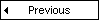# CSS Units### Measurements

Unit Description
% percentage
in inch
cm centimeter
mm millimeter
em 1em is equal to the current font size. 2em means 2 times the size of the current font. E.g., if an element is displayed with a font of 12 pt, then '2em' is 24 pt. The 'em' is a very useful unit in CSS, since it can adapt automatically to the font that the reader uses
ex one ex is the x-height of a font (x-height is usually about half the font-size)
pt point (1 pt is the same as 1/72 inch)
pc pica (1 pc is the same as 12 points)
px pixels (a dot on the computer screen)

### Colors

Unit Description
color_name A color name (e.g. red)
rgb(x,x,x) An RGB value (e.g. rgb(255,0,0))
rgb(x%, x%, x%) An RGB percentage value (e.g. rgb(100%,0%,0%))
#rrggbb A HEX number (e.g. #ff0000)